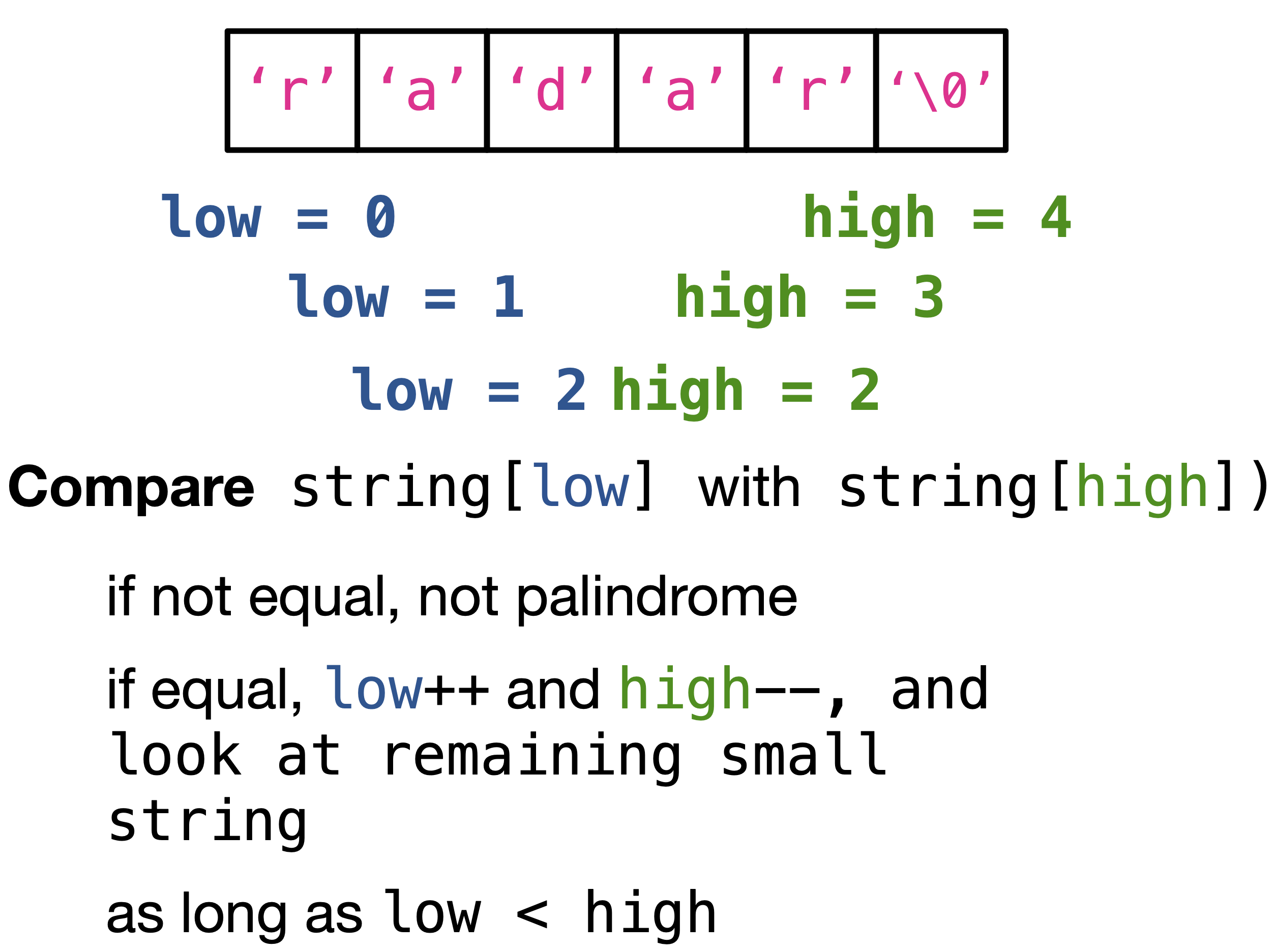# 11.3. Recursion in arrays#

In this chapter, we will learn how to use recursion to solve problems involving arrays, including strings.

## 11.3.1. Recursion on strings#

A string is an array of characters. To think of a string recursively, think of a string as

1. a character followed by a smaller string. For example, the string "hello" can be thought of as 'h' followed by "ello".

2. a character preceded by a smaller string. For example, the string "hello" can be thought of as "hell" followed by 'o'.

3. two characters enclosing a string. For example, the string "hello" can be thought of as 'h' followed by "ell" followed by 'o'.

The recursive call would be the same function called on the smaller problem/string, and the base case is when you have the smallest possible string, which is a string of length 1, or when you observe the '\0' at the end of the string.

## 11.3.2. Exercise: Is a String a Palindrome?#

Let’s write a recursive function to determine if a string is a palindrome, which is a string that reads the same forwards as backwards. For example, "radar" is a palindrome, but "hello" is not since "hello" is not the same as "olleh".

Think recursively! We need to compare the first and last characters of the string, and then compare the second and second-to-last characters of the string, and so on. With every iteration, the remaining characters in the string are two less than the previous iteration. Hence, in a recursive function, we need to compare the first and last characters of the string, and then call the function on the remaining characters in the string till there are not more characters to compare, which is the base case or terminating condition.

We show in the following figure that we start by comparing the character at index low with the character at index high. If they are not the same, we return false. If they are the same, we call the function on the remaining characters in the string, which is the string from index low + 1 to index high - 1. We should stop doing that when low >= high, which is the base case.Fig. 11.14 Steps to determine if a string is a palindrome.#

We can translate these steps to write the function as follows:

1bool isPalindrome(char *s, int low, int high) {
2  if (low >= high)
3    return true; // Base case: is a palindrome
4  else if (s[low] != s[high])
5    return false; // Base case: is NOT a palindrome
6  else // recursive call: compare the remaining characters from low + 1 to high - 1
7    return isPalindrome(s, low + 1, high - 1);
8}


Note that the function prototypes takes in the values of low and high to communicate the latest indices of the string that we are comparing. This function now has three parameters to pass, which is not ideal.

We can have a function with only one parameter that takes the string, and this function calls the function with three parameters. This function with three parameters is the recursive function that does the actual work.

The following code shows how the recursive function can be called from a function with only one parameter using a "helper" that takes in three parameters. Download isPalindrome.c if you want to run the program yourself.


#include <stdbool.h>
#include <stdio.h>
#include <string.h>

bool isPalindromeHelper(char *s, int low, int high) {
if (low >= high)
return true;
else if (s[low] != s[high])
return false;
else
return isPalindromeHelper(s, low + 1, high - 1);
}

bool isPalindrome(char *s) {
return isPalindromeHelper(s, 0, strlen(s) - 1);
}

int main(void) {
printf("isPalindrome(\"racecar\") = %d\n", isPalindrome("racecar"));
printf("isPalindrome(\"e\") = %d\n", isPalindrome("e"));
printf("isPalindrome(\"\") = %d\n", isPalindrome(""));
printf("isPalindrome(\"race\") = %d\n", isPalindrome("race"));
return 0;
}



More worked examples in-progress!

0 Questions

X